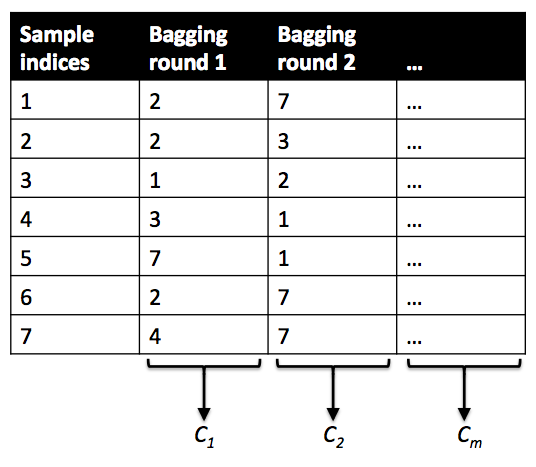# Difference between Random forest vs Bagging in sklearn

I know that Random forest uses Bagging, but how does ensemble give a single prediction in sklearn Bagging vs Random forest? Also, I do not understand why in Bagging you not need to choose a tree estimator, while not in RF? other than this, are the other steps the same? wht are main implementation differences in sklearn Bagging vs Random forest?

bonus points if you have "explain like I'm 5 years old" simple example using sklearn please.

• How many 5-year-olds write python scripts? – Sycorax Dec 14 '17 at 17:00
• Yeah, I did not understand that either. I kinda went with the spirit of what I thought he was asking. I think my answer is actually more 12-year-old-ish :-) – user188466 Dec 14 '17 at 18:20

we can use "Iris" dataset to demonstrate both Bagging and Random Forests

first, Let's take a look at the Iris dataset:

• 150 samples of flowers
• 3 classes $y$
• 4 continuous features $x_j$

to do example in sklearn, we need to import the usual suspects...

from sklearn.datasets import load_iris
from sklearn.ensemble import BaggingClassifier, RandomForestClassifier
import pandas as pd

y= pd.Series(iris.target)


then we specify which of two ML models we will be using:

# create Ensemble object/instance
model = BaggingClassifier(base_estimator=None)
model = RandomForestClassifier()


regardless of which of the 2 we use, our sklearn steps will be the same:

# Train the model using the training sets
model.fit(X_train, y_train)

# OUTPUT
## check score
model.score(X_train, y_train)

## Predict on test set
predicted= model.predict(X_test)


In order to visually and fully demonstrate the Bagging voting, WLOG we have a simplified version "Iris" dataset has:

• 7 total observations only
• 4 features $\mathbf{x}_j$
• 3 different possible species as the labels $y$
• instead of their Latin names, lets for simplicity denote the 3 different species as
• Species $\mathbf{A})$
• Species $\mathbf{B})$
• Species $\mathbf{C})$
• because sklearn needs numeric input always, their sklearn numeric labels $= [0, 1, 2]$
• Species $\mathbf{A}) \mapsto 0$
• Species $\mathbf{B})\mapsto 1$
• Species $\mathbf{C})\mapsto 2$for our 7-observation simplified "Iris" Training dataset, we:

1. Take 5 Bootstrap-samples

• each Bootstrap-sample is of cardinality 7 selected WITH replacement from our simplified "Iris" dataset

• i.e., each bootstrap-sample has 7 observations WITH possible Repetitions

then in step 2, we Train either:

• Train a distinct decision tree classifier on each of the Bootstrap-samples

• each tree is fit on its own distinct Bootstrap-sample of 7 observations
• Train a distinct Random Forests decision tree classifier on each of the Bootstrap-samples

• Set each Random Forests classifier to decision its tree node using only 3 of the 4 features $\mathbf{x}_j$
• the 3 features selected are a random subset of the 4 possible features $\mathbf{x}_j$
• (subset are drawn withOUT replacement)

so we end up with a Bootstrap-samples-aggregated ensemble of a total of 5 decision trees classifiers, which are either fully-grown in the case of Bagging or grown using feature subsets in the case of Random Forests.

1. Predict new data labels using Bootstrap-samples-aggregated ensemble meta-estimator
• Random Forests & other Bagging meta-estimators use all the 5 decision trees to predict the label $y$
• all 5 trees predict and RF meta-estimator averages their individual predictions
• (see example calculation below)

for a Test-set X_test consisting of a single previously-unseen flower:

if the $(A, B, C)$ predict_proba output of the 5 individual sklearn subestimator trees are:

1. $(0.75, 0.20, 0.05)$
2. $(0.60, 0.35, 0.05)$
3. $(0.55, 0.40, 0.05)$
4. $(0.35, 0.60, 0.05)$
5. $(0.50, 0.45, 0.05)$

then either of the Bootstrap-samples-aggregating meta-estimators' Test-set predict = A) on this specific flower-sample

• i.e., our new Test-set observation would be classified as Species A) by either the Bagging or Random Forests meta-estimator
• because the highest of the 3 classes' average predicted probability is for Species $\mathbf{A}) = \frac{3 \, = \sum bootstraps \,}{5 \; bootstraps} = 0.55$
• because all of sklearn's Bootstrap-samples-aggregating classification meta-estimators have a predict method whose averaging procedure returns the class with the highest average predicted probability

(and also to be able to use an image i found on the interwebs)

this (ridiculous) 7-datapoint"Iris" data set is for explanation purposes only

• Yes this is what I want. Essentially the difference between the two Sci-kit-learn models – MikeKim Dec 14 '17 at 4:19
• Btw I did not downvote you – MikeKim Dec 14 '17 at 4:31
• +1 Tried to up vote your answer, but I do not have enough points to do it. – MikeKim Dec 14 '17 at 6:31
• No worries. Please let me know if anything is unclear in my explanation/example. – user188466 Dec 14 '17 at 16:30

The difference is at the node level splitting for both. So Bagging algorithm using a decision tree would use all the features to decide the best split. On the other hand, the trees built in Random forest use a random subset of the features at every node, to decide the best split.